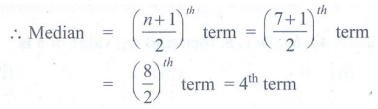Home | | Maths 7th Std | Exercise 5.3 (Median)

# Exercise 5.3 (Median)

7th Maths : Term 3 Unit 5 : Statistics : Median : Text Book Back Exercises Questions with Answers, Solution

Exercise 5.3

1. Fill in the blanks.

(i) The median of the data 12, 14, 23, 25, 34, 11, 42, 45, 32, 22, 44 is _________. [Answer: 25]

(ii) The median of first ten even natural numbers is ________. [Answer: 11]

2. Find the median of the given data: 35, 25, 34, 36, 45, 18, 28.

Solution:

Arranging the given data in ascending order 18, 25, 28, 34, 35, 36, 45.

Here the number of observations n = 7, which is odd.Median = ( [n + 1] / 2)th term = ([7 + 1] / 2)th term

= (8 / 2)th term = 4th term

Hence Median = 34

3. The weekly sale of motor bikes in a showroom for the past 14 weeks given below. 10, 6, 8, 3, 5, 6, 4, 7, 12, 13, 16, 10, 4, 7. Find the median of the data.

Solution:

Arranging the given data in ascending order 3, 4, 4, 5, 6, 6, 7, 7, 8, 10, 10, 12, 13, 16.

Here number of data n = 14, which is evenMedian = 1/2 {(n / 2)th term + (n / 2 + 1)th term

= 1/2 {(14 / 2)th term + (14 / 2 + 1)th term

= 1/2 {7th term + 8th term}

= 1/2 {7 + 7} = 1/2 (14) = 7

Median = 7

4. Find the median of the 10 observations 36, 33, 45, 28, 39, 45, 54, 23, 56, 25. If another observation 35 is added to the above data, what would be the new median?

Solution:

Arranging the given 10 observations in ascending order.23, 25, 28, 33, 36, 39, 45, 45, 54, 56. Here number of data n = 10, which is evenMedian = 1/2 {(n / 2)th term + (n / 2 + 1)th term

= 1/2 {(10 / 2)th term + (10 / 2 + 1)th term

= 1/2 {5th term + 6th term}

= 1/2 {36 + 39}

= 1/2 (75) = 37.5

Median = 37.5

If 35 is added to the above data then it will be the 5th term then number of data n = 11, odd

Median = ([n + 1] / 2)th term = ([11 + 1] / 2)th term

= (12 / 2)th term = 6th term

New median = 36

Objective type questions

5. If the median of a, 2a, 4a, 6a, 9a is 8, then find the value of a is

(i) 8

(ii) 6

(iii) 2

(iv) 10

Solution: a, 2a, 4a, 6a, 9a

Median = 4a = 8

a = 2

6. The median of the data 24, 29, 34, 38, 35 and 30, is_____________

(i) 29

(ii) 30

(iii) 34

(iv) 32

Solution: 24, 29, 30, 34, 35, 38

Median = [30 + 34] / 2 = 64 / 2 = 32

7. The median first 6 odd natural numbers is ________

(i) 6

(ii) 7

(iii) 8

(iv) 14

Solution: 1, 3, 5, 7, 9, 11

Median = [5 + 7] / 2 = 12 / 2 = 6

Exercise 5.3

1. (i) 25 (ii). 11

2. 34

3. 7

4. 37.5 ; 36

Objective type questions:

5. (iii) 2

6. (iv) 32

7. (i) 6

Tags : Questions with Answers, Solution | Statistics | Term 3 Chapter 5 | 7th Maths , 7th Maths : Term 3 Unit 5 : Statistics
Study Material, Lecturing Notes, Assignment, Reference, Wiki description explanation, brief detail
7th Maths : Term 3 Unit 5 : Statistics : Exercise 5.3 (Median) | Questions with Answers, Solution | Statistics | Term 3 Chapter 5 | 7th Maths